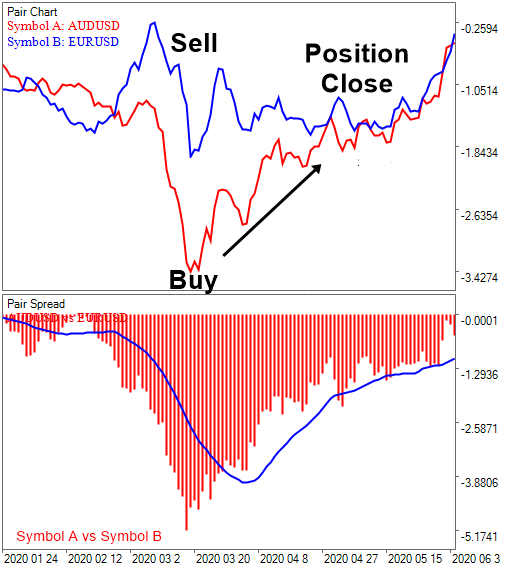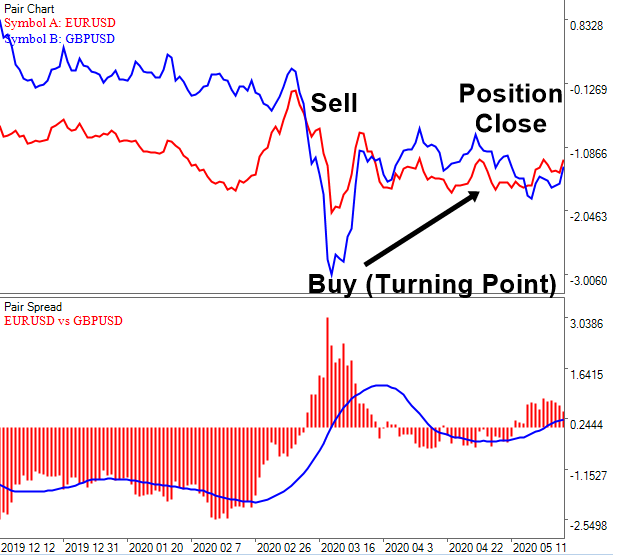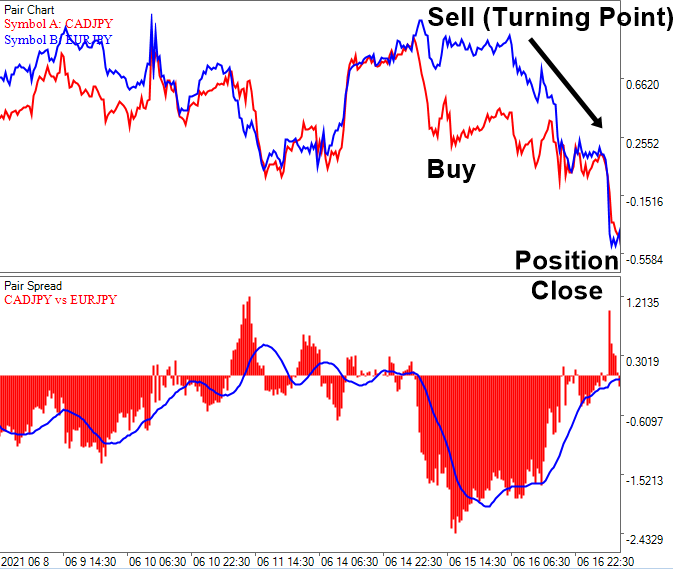# Turning Point in Pairs Trading

Pairs trading starts with identifying a pair of assets that are believed to have some long-run equilibrium relationship. When the spread has diverged sufficiently, the strategy will buy the undervalued asset and sell the overvalued asset. The strategy achieves a hedging or risk neutral state by taking two assets at the same time in different direction. Pairs trading strategy is applicable to both Forex and Stock market. The process of the pairs trading can be summarized into the following four steps.

1. Identify two assets whose prices have moved together historically in long term.
2. Calculate the correlation, co-integration, historical spread between two assets.
3. When the spread between two assets are diverged excessively high, then buy the undervalued asset and sell the overvalued asset.
4. When the spread is reverting back to normal range, close the positions of the two assets to take the profit.Figure 2-3: Normalized AUDUSD vs EURUSD on the top and spread chart on the bottom (Chart: www.algotrading-investment.com, Optimum Chart)

Since the pairs trading involves to take the positions for two assets, this provides some of its own properties, which is different from other strategy. For example, we could provide two illustrative examples when the positions are closed with profits. These two illustrative examples are not necessarily the exhaust list of scenarios for the movements of two assets. However, we have selected them as they can visualize the process of the formation of the turning point better than other scenarios.

For the first example, consider that we bought the one asset with low price and sold another asset with high price when the spread was diverged sufficiently high. In this example, the asset with low price (=Symbol B) could make the bullish turning point to reach the price level of another asset because they are supposed to move together in long run. We are in profit due to the sharp rise in the asset with low price (=Symbol B) after the bullish turning point.Figure 2-4: Normalized EURUSD vs GBPUSD on the top and spread chart on the bottom (Chart: www.algotrading-investment.com, Optimum Chart)

In the second example, the asset with high price (=Symbol B) could make the bearish turning point to reach the price level of another asset because they are supposed to move together in long run. We are in profit due to the sharp fall in the asset with high price (=Symbol B) after the bearish turning point.Figure 2-5: Normalized CADJPY vs EURJPY on the top and spread chart on the bottom (Chart: www.algotrading-investment.com, Optimum Chart)

From the two illustrative examples, we could draw two useful trading principle. Firstly, when the spread is sufficiently high, it is possible to have a turning point between two assets. The bullish turning point could happen to the asset with low price or the bearish turning point could happen to the asset with high price. As in value investing, the pairs trading provides an explanation to the formation of the turning point in the financial market. Secondly, taking the opposite positions on two assets reduce the risk of the market volatility. This is considered as the hedging or the risk neural strategy. Since we are holding one position in opposite direction to other position, we are exposed less on the market volatility. Although hedging is an optional practice only, you never know if you like or dislike such a trading principle. If you do not understand the hedging effect, you might open a Forex demo or simulation account for an educational purpose. Search two highly correlated assets. Then buy one and sell the other. Monitor the profits and loss of the two assets for some time.

This article is the part taken from the draft version of the Book: Science Of Support, Resistance, Fibonacci Analysis, Harmonic Pattern, Elliott Wave and X3 Chart Pattern. Full version of the book can be found from the link below: